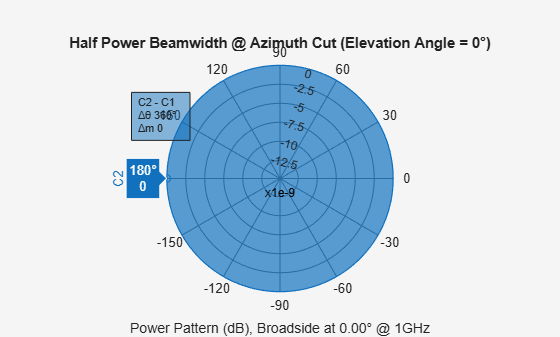# beamwidth

Compute and display beamwidth of sensor element pattern

Since R2020b

## Syntax

``beamwidth(element,freq)``
``beamwidth(element,freq,Name,Value)``
``[bw,angles] = beamwidth(___)``

## Description

example

````beamwidth(element,freq)` plots the 2-D power pattern (in dB) of the `element` for all azimuth angles at an elevation angle of zero degrees. The plot displays the half-power beamwidth (in degrees) at the frequency specified in `freq` (in Hz) and the angles (in degrees) in azimuth at which the magnitude of the power pattern decreases by 3 dB from the peak of the main beam.```
````beamwidth(element,freq,Name,Value)` plots the beamwidth with the specified parameter `Name` set to the specified `Value`. You can specify additional name-value pair arguments in any order as (`Name1,Value1,...,NameN,ValueN`).Example: `beamwidth(element,1e9,'Cut','Elevation)````

example

````[bw,angles] = beamwidth(___)` returns the angular beamwidth `bw` (in degrees). The function also returns the corresponding angle values (in degrees) that mark the beamwidth. ```

## Examples

collapse all

Plot the beamwidth for an isotropic antenna at 1 GHz.

Create an isotropic antenna using a `phased.IsotropicAntennaElement` object.

`antenna = phased.IsotropicAntennaElement('FrequencyRange',[800e6 1.2e9])`
```antenna = phased.IsotropicAntennaElement with properties: FrequencyRange: [800000000 1.2000e+09] BackBaffled: false ```

Using the `beamwidth` function, plot the half-power (3 dB) beamwidth for the antenna. Use a frequency value of 1 GHz.

`beamwidth(antenna, 1e9)````ans = 360 ```

Calculate the half-power beamwidth and angles of a cosine antenna element.

Create a `phased.CosineAntennaElement` object with the `'CosinePower'` exponents set to 10.

`myAnt = phased.CosineAntennaElement('CosinePower',[10 10])`
```myAnt = phased.CosineAntennaElement with properties: FrequencyRange: [0 1.0000e+20] CosinePower: [10 10] ```

Compute the beamwidth and angles of the antenna element when it is operating at 1 GHz. Set `'dbDown'` to 3 dB.

`[BW,Ang] = beamwidth(myAnt,1e9,'dbDown',3)`
```BW = 29.9600 ```
```Ang = 1×2 -14.9800 14.9800 ```

## Input Arguments

collapse all

Antenna or microphone element, specified as a Phased Array System Toolbox System object.

Frequency used to calculate the beamwidth, specified as a scalar in Hz.

Example: `1e9`

Data Types: `double`

### Name-Value Arguments

Specify optional pairs of arguments as `Name1=Value1,...,NameN=ValueN`, where `Name` is the argument name and `Value` is the corresponding value. Name-value arguments must appear after other arguments, but the order of the pairs does not matter.

Before R2021a, use commas to separate each name and value, and enclose `Name` in quotes.

Example: `beamwidth(element,1e9,'Cut','Azimuth','CutAngle',45)` plots the beamwidth of the antenna element that is operating at a frequency of 1 GHz, with the slice direction set to `'Azimuth'`, and the cut angle set to 45 degrees.

The slice direction in azimuth-elevation space along which the beamwidth is computed, specified as the comma-separated pair consisting of `'Cut'` and `'Azimuth'` for the azimuth plane, and `'Cut'` and `'Elevation'` for the elevation plane.

Corresponding angle (in degrees) for the plane to get the required 2-D cut, specified as the comma-separated pair consisting of `'CutAngle'` and a scalar. If `'Cut'` is specified as `'Azimuth'`, then `'CutAngle'` (Elevation) should lie between [−90, 90] degrees. If `'Cut'` is specified as `'Elevation'`, then `'CutAngle'` (Azimuth) should lie between [−180, 180] degrees.

Data Types: `double`

Power value from the peak of the main lobe, specified as the comma-separated pair consisting of `'dBDown'` and a positive scalar. The default value of 3 dB is equivalent to half-power beamwidth. To calculate the first-null beamwidth, specify the `'dBDown'` value as `Inf`. Units are in dB.

Data Types: `double`

## Output Arguments

collapse all

Angular beamwidth of the sensor element, returned as a scalar in degrees.

Data Types: `double`

Angle values of the beamwidth, returned as a 1-by-2 vector. The two elements in the vector [amin, amax] define the beamwidth `bw` as amaxamin.

## Version History

Introduced in R2020b